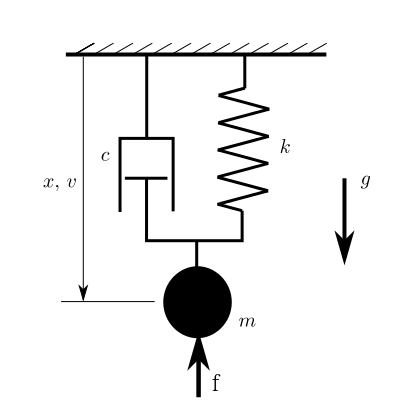# [PYTHON] Equation of motion with sympy

## Equation of motion with sympy

It seems that sympy, a computer algebra library in python, can handle equations of motion that appear in physics, so I would like to try it. This time, I will write a script to derive the equation of motion of the mass-spring-damper system, which is also in pydy_example on the reference site. First, suppose you have a mass spring damper system as shown in the figure. If you formulate the equation of motion normally, it will be as follows.Equation of motion

{\bf M}\dot{{\bf x}} = {\bf f}({\bf x})


here

{\bf x} = [{\it x}, \dot{{\it x}}]^T \\
{\bf M} = \begin{bmatrix} 1 & 0 \\ 0 & m \end{bmatrix} \\
{\bf f}({\bf x}) = \begin{bmatrix} \dot{x} \\ -k x - c \dot{x} + m g + f \end{bmatrix}


Suppose that Next is the script. We use a module called sympy.physics.mechanics. I'm using the Kane's method to formulate the equation of motion, but I'm not sure about this. There seems to be a way to solve it with the Lagrange method.

#### mass_spring_dumper.py


#!/usr/bin/python
#coding:utf-8
import sympy as sym
import sympy.physics.mechanics as me

x, v = me.dynamicsymbols('x v')
m, c, k, g, t, f = sym.symbols('m c k g t f')

#Creating a coordinate system
ceiling = me.ReferenceFrame('C')

o = me.Point('o') #Ceiling point
p = me.Point('p') #Mass point
o.set_vel(ceiling, 0)
p.set_pos(o, x * ceiling.x)
p.set_vel(ceiling, v * ceiling.x)

#Calculate the external force applied to the mass point
damping = -c * p.vel(ceiling) #Dumping
stiffness = -k * p.pos_from(o) #Spring
gravity = m * g * ceiling.x #gravity
exf = f * ceiling.x #Other external forces

forces = damping + stiffness + gravity + exf
print forces

mass = me.Particle('mass', p, m)
kane = me.KanesMethod(ceiling, q_ind=[x], u_ind=[v], kd_eqs=[v - x.diff(t)])
kane.kanes_equations([(p, forces)], [mass])
M = kane.mass_matrix_full
f = kane.forcing_full
print M
print f
print M.inv() * f


The output looks like this:

(-c*v + f + g*m - k*x)*C.x
Matrix([[1, 0], [0, m]])
Matrix([[v(t)], [-c*v(t) + f + g*m - k*x(t)]])
Matrix([[v(t)], [(-c*v(t) + f + g*m - k*x(t))/m]])


If you learn how to use it, it will be a very powerful tool. There are maple and mathematica for a fee, but it is expensive to use such software for free, so I'm grateful that such software can be used for free.

## Reference site

sympy documentation http://docs.sympy.org/latest/index.html pydy examples https://github.com/PythonDynamics/pydy_examples